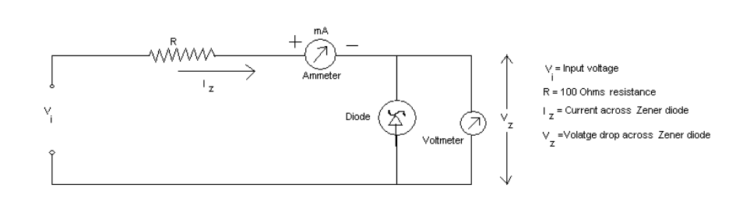## Performing the Experiments Using the PSLab Android App

General laboratory experiments can be performed using core functionalities offered by the PSLab hardware device like Programmable Voltage Sources, Programmable Current Source, Analog to Digital Converter, Frequency Counter, Function Generators, etc. In this post we will  have a brief look on a general laboratory experiment and how we can perform it using the  PSLab Android App and the PSLab hardware device.

We are going to take Zener I-V Characteristics Curve experiment as an example to understand how we can perform a general experiment using the PSLab device. First, we will  look at the general laboratory experiment and it’s format. Then we will see how that experiment can be performed using the PSLab Android App and the PSLab Hardware Device.

#### Experiment Format of General Experiment in Laboratory

AIM: In this experiment, our aim is to observe the relation between the voltage and the corresponding current that was generated. We will then plot it to get the dependence.

Apparatus:

• A Zener Diode
• A DC Voltage Supplier
• 100 ohm resistor
• 2 multimeter for measuring current and voltages
• Connecting wires

Theory: A Zener Diode is constructed for operation in the reverse breakdown region.The relation between I-V is almost linear in this case, Vz = Vz0 + Iz * Rz , where Rz is the dynamic resistance of the zener at the operating point and Vz0 is the voltage at which the straight-line approximation of the I-V characteristic intersects the horizontal axis. After reaching a certain voltage, called the breakdown voltage, the current increases drastically even for a small change in voltage. However, there is no appreciable change in voltage accompanying this current change. So, when we plot the graph, we get a curve which is very near to the x-axis and nearly parallel to it until a particular potential value, called the Zener potential, is reached. After the Zener potential Vz value, there will be a sudden change and the graph becomes exponential.

Procedure: Construct the circuit as shown in figure belowNow, start increasing the voltage until a reading in the multimeter for current can be obtained. Note that reading. Now, start increasing the input voltage and take the corresponding current readings. Using the set of readings observed,  construct a V vs I graph. This graph gives us the I-V characteristics. The slope of the curve at any point gives the dynamic resistance at that voltage.

Result: The Characteristic curve has been verified after plotting V-I data points on the graph.

#### Experiment format in PSLab Android App

We have a ViewPager that renders two fragments:

1. Experiment Doc– It consists of information like the Aim of experiment, Schematic, Output screenshot that we will get after the experiment has been performed.
2. Experiment Setup– It consists of the setup to configure the PSLab device. This fragment is analogous to the experiment apparatus of the laboratory.

Below is a gif showing the experiment doc of the Zener I-V experiment which is to be performed using the PSLab device. It consists of a schematic and a screenshot of the output that we get after performing the experiment.

Make the circuit connections on a breadboard as shown in the schematic. After the circuit is complete we need to configure experiment.

To configure the experiment, we give the initial voltage, the final voltage and the step size. After clicking on START EXPERIMENT, the voltage is varied on the PV1 channel from the initial voltage to final voltage by increasing the voltage in step size. At each variation of voltage, the current is calculated by dividing the voltage difference between resistor by its resistance value i.e

`I = ( VPV1 - VCH1 ) / R`

As soon as the initial voltage reaches the final voltage, the experiment stops and data points are plotted on the graph. From the graph we can see the change in the current through a zener diode when the voltage varies across it’s terminals.

The output that was obtained after the experiment is I-V characteristic curve for Zener Diode as shown in the image below.It can be clearly seen that after the breakdown voltage (~0.7V) the  current increases drastically with respect to the  increase in the voltage. After this point, the voltage can be considered  nearly constant unlike the current which varies exponentially.

In the PSLab Android App, there are read-back errors while reading bytes serially from the PSLab Hardware Device. As a result, the data points are not read accurately and an inaccurate plot is generated on the graph as shown in the image below.